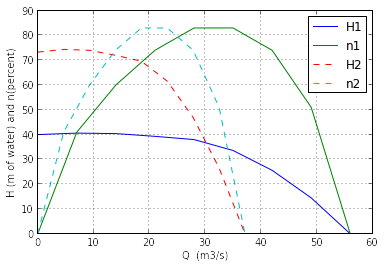# Chapter 23: Performance of Rotodynamic Machines¶

## Example 23.1, Page 814¶

In :
from __future__ import division
import math
from pylab import *
%matplotlib inline

#Initializing  the  variables
Q  =  []
for c in range(9):
Q.append(7*c)
H  =  [40, 40.6, 40.4, 39.3, 38, 33.6, 25.6, 14.5, 0];
n  =  [0, 41, 60, 74, 83, 83, 74, 51, 0];
N1  =  750;
N2  =  1450;
D1  =  0.5;
D2  =  0.35;

#Calculations
Q2=[]
H2=[]
for c in range(9):
Q2.append(Q[c]*(N2/N1)*(D2/D1)**3);
H2.append(H[c]*(N2/N1)**2*(D2/D1)**2);

plot(Q,H,label='H1')
plot(Q,n,label='n1')
plot(Q2,H2,'--',label='H2')
plot(Q2,n,'--',label='n2')
legend( loc='upper right', numpoints = 1 )
xlabel("Q  (m3/s)");
ylabel("H (m of water) and n(percent)")
grid(True)
show()## Example 23.2, Page 831¶

In :
from __future__ import division
import math

#Initializing  the  variables
n  =  0.9;
g  =  9.81;
D  =  1.45;
N  =  375/60;
H  =  200;                                                                #  Real  height
x  =  165;                                                                #  Theta
P  =  3750*10**3;
rho  =  1000;

#Calculations
v1  =  (2*g*h)**0.5;
u  =  math.pi*D*N;

P_b  =  P/n_a;
ppj  =  P_b/2;                                                        #  Power  per  jet
d  =  (8*ppj/(rho*math.pi*v1**3))**0.5  ;
print "the efficiency of runner :",round(n_a,3)
print "Diameter of Jet (m)      :",round(d,3)

the efficiency of runner : 0.933
Diameter of Jet (m)      : 0.156


## Example 23.3, Page 834¶

In :
from __future__ import division
import math
#Example  23.3

#Initializing  the  variables
g  =  9.81;
H  =  12;
n  =  0.8;
w  =  300*2*math.pi/60;
Q  =  0.28;

#Calculations
V_f1  =  0.15*(2*g*H)**0.5;
V_f2  =V_f1;
V_w1  =  (n*g*H)**0.5;
u1  =  V_w1;
theta  =  math.atan(V_f1/u1);
u2  =0.5*u1;
B2  =  math.atan(V_f2/u2);
r1  =  u1/w;
b1  =  Q/(V_f2*0.9*2*math.pi*r1);                          #  vanes  occupy  10  per  cent  of  the  circumference  hence  0.9
b2  =  2*b1;

print "Guide vane angle    (degree)  :",round(theta*180/math.pi,2)
print "Vane angle at exit  (degree)  :",round(B2*180/math.pi,2)
print "Width of runner at inlet (mm) :",round(b1*1000,1)
print "Width of runner at exit  (mm) :",round(b2*1000,1)

Guide vane angle    (degree)  : 13.34
Vane angle at exit  (degree)  : 25.38
Width of runner at inlet (mm) : 69.6
Width of runner at exit  (mm) : 139.3


## Example 23.4, Page 838¶

In :
from __future__ import division
import math
import sympy
from sympy import solve,symbols

#Initializing  the  variables
H  =  35;
g  =  9.81;
D  =  2;
N  =  145/60;
z  =  30*math.pi/180;                          #  angle  between  vanes  and  direction  of  runner  rotation
y  =  28*math.pi/180;                          #  angle  between  runner  blades  at  the  outlet.

#Calculations
H_net  =  0.93*H  ;                            #  since  7%  head  is  lost
v1  =  (2*g*H_net)**0.5;
u  =  math.pi*N*D;

# from inlet velocity triangle
V_r1=14.3
## ash = cos(beta1+z)
ash = (V_r1**2+v1**2-u**2)/(2*V_r1*v1)
beta1=(z+math.acos(ash))*180/math.pi  # in degrees

V_r2=(1-8/100)*V_r1         #8 % loss due to friction
V_w2= u - V_r2*math.cos(y)

E  =  (u/g)*(V_w1  -  V_w2);
n  =  E/H;
print "Blade angle at inlet :",round(beta1,1)
print "Efficiency    (%)    :",round(n*100)

Blade angle at inlet : 62.1
Efficiency    (%)    : 81.0


## Example 23.5, Page 844¶

In :
from __future__ import division
import math

#Initializing  the  variables
s  =  0.03;
P  =  185*10**3;
rho  =  0.86*10**3;
A  =  2.8*10**-2;
N  =  2250/60;
D  =  0.46;

#Calculations
R0  =  0.46/2;
Ws_Wp  =  1-s;
n  =  Ws_Wp;
Pf  =  s*P;
Q  =  (2*Pf*A**2/(3.5*rho))**(1/3);
Wp  =  2*math.pi*N;
Ri  =  ((1/Ws_Wp)*(R0**2  -P/(rho*Q*Wp**2)))**0.5;#  Modified  equation  for  power  transmission.
Di  =  2*Ri;
T  =  P/(rho*Wp**3  *D**5);

print "Mean diameter (mm) :",round(Di*1000)
print "Torque Coefficient :",round(T,4)

Mean diameter (mm) : 326.0
Torque Coefficient : 0.0008